## Friday, August 30, 2013

### R1.2: Probability Distributions - Calculations for Statistical Distributions in R

In this post we will explore the different calculations we can apply on statistical distributions in R. We will cover:

### 1. Density and Point Probability

When we have a continuous distribution, such as a normal distribution (Gaussian), the density is a measure of the relative probability of getting a value close to x. So for a particular interval in the distribution, the probability of getting a value in the interval is the area under the curve.

For example, we can use the familiar 'bell-curve' otherwise known as a normal distribution. Through the console in R, we will create a plot showing the density of a normal distribution: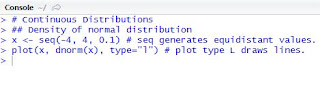Fig. 1: Creating a Density Plot for a Normal Distribution
For the x values, I used a sequence function where it repeats values from -4 to 4 in increments of 0.1. The y values are obtained with the dnorm function, which results in the density of a normal distribution given the x values. To make the plot visually pleasing, the plot type is changed to "l" for lines, for which we get: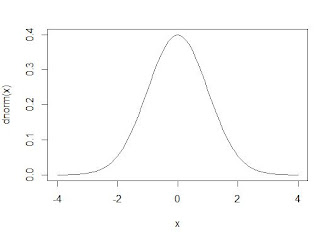Fig. 2: Density plot of a Standard Normal Distribution
Shifting to discrete distributions, such as binomial distributions, the variables can only take distinct values, such as number of iPads sold. The number of iPads are distinct, because you cannot sell half an iPad, but only in whole quantities, such as: one, twelve, one hundred, etc. This is distinct from continuous where the variables can any value within the specified range, such as temperatures or mass. A binomial distribution is a special case of discrete distributions, where the outcomes are yes/no, success/failure, or of two possibilities. So for this binomial distribution, we will suppose number of independent trials at n = 50, and a probability of 'success' at 0.33.Fig. 3: R Code for Plotting a Binomial Distribution
Like the plotting the normal distribution, the y values in plotting the binomial distribution is given by dbinom(x, size=50, prob=0.33). The size corresponds to the n of 50, and the prob represents the probability of success at 0.33.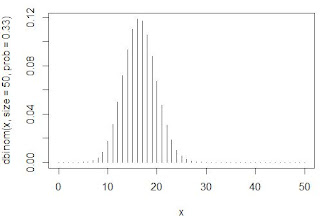Fig. 4: Plot of a Binomial Distribution, Histogram Type
Above in Figure 4, we see the plot of the binomial distribution with n = 50 and a probability at 0.33. We see the highest point probabilities centered around 16 and 17, where they have the highest chances of occurring. The chances of getting successes lower and higher than 16 or 17 (moving away from 16 or 17) drop as the point probabilities lower. This makes sense, as the probability was set at 0.33 with a size of 50 (0.33*50=16.5), and as this is a discrete distribution with whole success values so they must be highest around 16 and 17.

### 2. Cumulative Probability

Cumulative probability distributions express the probability of 'hitting' a specified 'x' or less in a given distribution. I will not show plotting cumulative probability distributions, because most of the time, actually numbers are desired. So if we have a non-standard normal distribution of blood pressures with a mean of 132 and a standard deviation of 13. Suppose we encounter a patient with a blood pressure of 160 (systolic, hopefully). Our 'x' in this situation is 160, so what is the probability of patients with a blood pressure of 160 and less?Fig. 5: Cumulative Probability of a Normal Distribution with u=132 and sd=13
The pnorm function gives us the cumulative probability in a normal distribution. From Figure 5, we see that 98.44% of patients have a blood pressure of 160 and lower. If we subtract it from 1, we could observe that 1.56% of patients have a blood pressure of 160 or higher.

For discrete distributions, the cumulative probability holds the similar, as we use the
pbinom function. Suppose we have 20 people choosing between Coke (A) and Pepsi (B), and say the preference is equal (p=0.5) due to a blind test. In the test, 16 people chose option A, Coke. How likely is it that Coke was chosen by 16 people over Pepsi?Fig. 6: Cumulative Probability of a Binomial Distribution with n=20 and prob=0.5
Using the pbinom function, with size being the number of people and prob being the probability of the first option, we see that we have a 99.87% probability that 16 people or less would choose Coke. What is the probability of getting 16 or more people? that would be using pbinom at 15, instead of 16 because we want to include 16 when we subtract the probability from 1. So chances of 16 or more people choosing Coke over Pepsi is 0.59%.

But what if we did not know which was better before the test, then we require adding the probability of extreme results going the other way. So we would include the chances of 4 or less people choosing option A (Coke) over B (Pepsi).Fig. 7: Cumulative Two-Tailed Probability of a Binomial Distribution, n=20, prob=0.5
While incorporating the probability of 16 or more in Figure 6, we would also include the the probability of 4 or less as shown in Figure 7. The two-tailed probability of 16 people choosing option A out of 20 people with a probability of 0.5 is 1.18%, which is twice the one-tailed probability.

### 3. Quantiles Quantiles are the inverse of cumulative distribution functions, because a p-quantile finds the value where there is probability p of getting the value less or equal to it. So the median, is the value at which the distribution has half of its values less than it, also known as the 50% quantile. In the back of statistics books, you most likely will find fixed sets of probabilities tables that show the boundary at which a test statistic must pass to become  significant at that level. They can range from 90%, 95%, or 99%, with the most often used at 95% level.Fig. 8: Formula for the 95% Confidence Interval of a Normal Distribution
Here in Figure 8, we have the formula for a 95% confidence interval (CI). Given the vitals of a normal distribution, x bar (sample mean), sigma (standard deviation), n (sample size), we can derive the 95% CI for the u, which is the true mean. Suppose x bar= 83, sigma = 12, and n = 5 (small but okay). The standard error of the mean (sem) is the normalized factor adjusting the quantile coefficient (Figure 10) in the formula (Figure 8).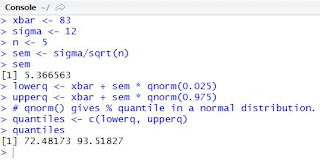Fig. 9: Code for Standard Error of the Mean and 95% CI
In the code above, we see that the sem (5.367) is calculated by dividing the standard deviation by the square root of the sample size. The sem is then multiplied by the 0.025 and 0.975 quantiles (so there is 2.5% on each tail) then added to the xbar to get the confidence interval about the true mean. So with the lower and upper 95% confidence interval of this sample, we are certain that 72.48 and 93.52 covers the true mean.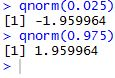Fig. 10: qnorm Functions and Quantile Values for Standard Normal Distribution
On a side note, the qnorm(k) function gives us the k-quantile value in a standard normal distribution where the % of values in the distribution is less than or equal to k. The 2.5% and 97.5% quantiles you will see often in statistics, which is usually abbreviated at -1.96 and 1.96, respectively. Between the 2.5% and 97.5% quantiles lies 95% of the values, hence, 95% confidence interval.

Here is some extra code for some fun:Fig. 11: Z Values, and 95% CI Calculation
What we have here in Figure 11 is the deconstruction of the 95% CI values into the Z scores, which is the standard 95% quantiles at -1.96 and 1.96. As you can see, the square root of n divided by the standard deviation (opposite of sigma/sqrt(n)) is multiplied to the difference of the quantile value and the xbar. Next we have the concatenation of the 95% quantiles and using that to multiply the standard error of the mean to put the lower and upper quantiles together.

### 4. Pseudo-random Numbers

Random numbers drawn from R do not seem random at all; they are set by seed numbers, that is why they are called pseudo-random. These generated random numbers behave for practical purposes, as random. So they can be used to simulate sets of 'randomly' generated numbers.Fig. 12: Randomly Generated Sample Distributions
In the sample distributions shown in Figure 12, we observe that the first two are two sets of 10 randomly generated numbers of a normal distribution. However, the numbers are different because the seed number is continually being used unless told to be reset, so the randomly generated numbers will not (likely) be the same. This shows that even when we generate a normal distribution randomly, we will not get the same values, which proves to be practical.

Secondly, we can also adjust the arguments in the random distribution functions, such as adjusting the mean to 7, and standard deviation to 5, or setting the success of a binomial distribution at 10 with a sample size of 20 and a probability of success at 0.5.

There are many more types of distributions and statistical tests available in R, which build on the fundamental calculations on distributions in this post. We will explore these in later posts.

Wayne

## Thursday, August 29, 2013

### R1.1: Probability Distributions - Random Sampling, Combinatorics in R

In this post we will work with R to perform some statistical tests and sampling using probability distributions.

Not all data sets have exact distributions that match a specific probability density function. There is a certain degree of randomness and 'un-reproducibility' to it. For example, ask 100 people with iPads how many times they use their iPads to view their Twitter feeds, and we will get a set of seemingly random numbers. That is to say, the data is almost guaranteed not to fit a perfect statistical model, such as a normal distribution or a Poisson distribution. However, these theoretical statistical distributions can enable us to have a better understanding and view of the data we work with. Let us dive in!

### 1. Random Sampling

In R we can draw random samples from a specific distribution. A basic example would be picking numbers for the Powerball lottery. To get the jackpot, we would have to match all six numbers on our \$2 ticket, with five of them ranging from 1 to 59, and the last red ball ranging from 1 to 35 (they are drawn from two separate sets of balls). Here is the drawing of the 'staggering' \$217.2 million Powerball jackpot in February 2013:

The Powerball site gives the odds of winning the jackpot at dismal 1 in 175,223,510 (I do not like it either, but hey, a ticket is only two dollars). We will derive this number further into the post.

To simulate this in R, we can choose sample numbers. The code for the five white balls is followed by the code for the red powerball, with results shown.Fig. 1: Powerball Number Sampling
The the sample function picks a specified number of samples (5 and 1) from a range of numbers (1 to 59 and 1 to 35). We can concatenate the two sets of numbers together with the concatenate function and assign the result to pbdrawing.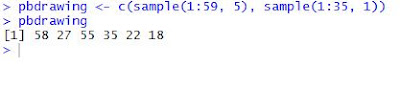Fig. 2: All Six Numbers in a Sample Ticket
You might notice that the numbers 'drawn' the second time in Figure 2 were different than in Figure 1. This is because every time we use the sample function, we get a new set of sampled numbers. However, since we stored them into pbdrawing, the numbers in there will stay the same (unless we assign different numbers to it after).

The default for the sample function is not to replace the values selected. The Powerball numbers are not replaced in the actual drawing. We can tell R to replace by adding a third argument, replace=True. This would be applicable in coin tossing. Say we toss a coin 10 times, assuming equal probability. Just because we toss a heads does not mean we cannot obtain that result again. That is when we set replace to True.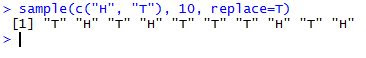Fig. 3: Results of Ten Coin Tosses
As you can see, we get 6 tails and 4 heads from 10 tosses. The result is not split down the middle, because it is random  sampling. If we would like to split the probability unevenly, we can alter the probability of the events in the sample range we are picking from. Suppose we have an electronic prototype, say the next generation iPads, which has a 10% screen failure rate after a month of testing (ouch, glad it is still a prototype). We can take a random sample of 10 next-gen iPads and see how many screens failed at the end of the testing period.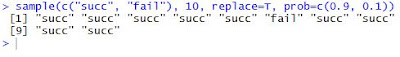Fig. 4: Successes and Failures
We represented the good iPads by "succ", short for success, and the iPads with bad screens with "fail". The additional code redefines the probability to be 0.9 for the first outcome (good screen!), and 0.1 (or 10%) for the second outcome of screen failure. So we have 9 iPads with good screens and 1 with a bad screen, which is about what we could expect in a random sample of 10 iPads.

### 2. Probability Calculations & Combinatorics

Let us go back to the Powerball example using combinatorics. To recap, Powerball drawings occur from drawing 5 numbers from balls labeled 1 through 59 and one red ball from balls labeled 1 through 35.

If we draw the first five white balls, how many different permutations would be possible? That is, how many different ways can we draw the 5 numbers out of the 59? Suppose we take into account the order of the numbers. Then we would calculate the permutation using factorials (where 4! is 4*3*2*1).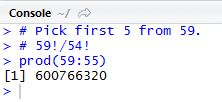Fig. 5: Permutation of Picking 5 from 59 numbers
The number of ways we can pick from 59 white balls is 59, and after the first pick, we have 58 left (no replacement). And choosing from the 58 there are 58 different balls, etc. So we need 59*58*57*56*55. We can write it using factorials: 59!/54! works because the numbers 54 and below in the denominator cancel out the numbers 54 and below in the numerator. Therefore we are left with: the product of numbers 59 to 55, as prod(59:55)as shown in Figure 5. R tells us that there are 600,766,320 ways (in order) we can pick 5 numbers from 59 balls.

But in the Powerball lottery, it does not matter what order the numbers are picked (like in the YouTube video), you just need to match them. So order does not matter; and to calculate how many ways we can choose 5 numbers from 59 balls in any order, we need to understand combinations.

We start with the permutation we calculated previously. That large number (6 hundred million+), is the number of ways we can choose 5 numbers in unique order. If order does not matter, the number of ways will be less. So we can simply divide it with the number of ways the 5 chosen numbers can be ordered. The first lucky number has 5 locations, 1st, 2nd, 3rd, 4th, or 5th one chosen, and the second number has 4 locations, etc. Therefore, we take 5! for the number of balls we picked, and use it to divide the permutation.Fig. 6: Combination of White Powerball Numbers
In Figure 6, we see the permutation, followed by the combination calculation, and then by the handy R function for combination, the choose function. We observe the same number of ways for the choose function and our manual calculation derived from permutation.

The mathematical formula for combination (also known as the binomial coefficient), where n is the number of elements and k is the number of picks: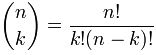Fig. 7: Number of k-Combinations in n-Elements
and is commonly read as "n choose k" (hence the name of the R function).

So when choose the final red ball from 35 balls, there is 35 different balls to choose from. We can apply the formula (however obvious the solution) to verify: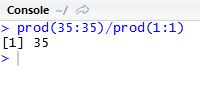Fig. 8: Combinations of the Red Ball
Needless to say, the 34! cancels out all but the 35 in the 35! and 1! is simply 1, so the result is 35 different ways to choose a red ball from 35 of them. Now with different sets of balls, multiplying the number of ways to choose the white ones and the red one will give us the number of possible ways the Powerball lottery can draw its numbers.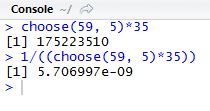Fig. 9: Combination of White Balls and Red Ball with Probability
As we can see, the result of 175,223,510 is the number of different combinations of Powerball ticket numbers. This matches the odds of winning the jackpot, as given on their website. The probability, frankly, does not look good. At all. It looks like we have a 0.000000571% chance of winning the jackpot with any random ticket (that is a lot of zeros).

However you have to play for a chance of winning (a ticket to dream, in my opinion). So best of luck when playing Powerball!

In the next post for Probability Distributions in R, we will cover calculations from different probability distributions.

-Wayne

## Friday, August 23, 2013

### P3.2: Beginning DAX 2

And we are back again for more DAX! This post is a continuation in the understanding DAX series (link to P3.1.) Here we will gain a deeper understanding of calculated columns and fields (introduced in P2.2) from a DAX perspective.

### Understanding Calculated Columns & Fields

Calculated columns and calculated fields are actually quite different despite initial similarities. Here we will explore the differences of columns of fields, while gaining DAX concepts and syntax.

#### Calculated Columns

Before in P2.2, we touched upon the notion of creating a calculated column and a calculated field. By clicking the Add Column button on the Design tab, we can create a calculated column in PowerPivot. It is versatile in PivotTables; also the DAX expression for the column applies to the current row of the table. When we reference a column, it returns the value of that particular column in that specific row.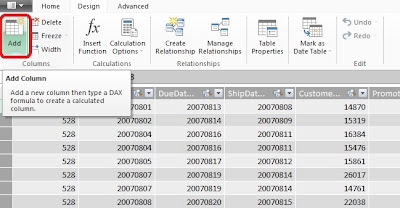Fig. 1: Add Column Button
Note:
There are DAX functions which aggregate the values of a column for a whole table. To evaluate a subset of rows, we use DAX to specify a range of rows or a single value from another row and operate on that subset table. Essentially we can restrict rows and apply filters so the desired rows are selected for computation.

#### Calculated Fields

Now for something a little different. While calculated fields use the same DAX syntax as calculated columns, calculated fields evaluate the expression at the context of the cell of the PivotTable, not at the row level of the PowerPivot table like a calculated column.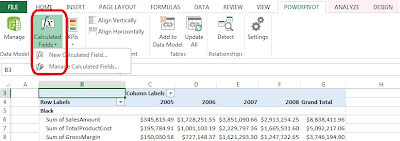Fig. 2: Calculated Fields Button
Which is why we arrived at a wrong result when we tried to calculate the gross margin as a percentage of the sales as a calculated column. In fact, PowerPivot calculated the gross margin percentage for each row using that method. What we wanted was to divide the gross margin by the sales within the context (column and row designation) of the PivotTable; we did not want each percentage in each row added, we required the gross margin percentage of the total (in say, Year 2006.)

So if we used the regular formula to calculate a field, we could get the following error:Fig. 3: Calculated Field Error Using the Same DAX Expression as Calculating a Column
That is because the GrossMargin column has a different value in each row, and we would rather see the aggregate of the GrossMargin divided by the aggregate SalesAmount for each cell context in the PivotTable.

The correct method was to type in the sum of each:
=SUM(Sales[GrossMargin]) / SUM(Sales[SalesAmount])
and then divide them, as shown in Figure 4.Fig. 4: Using the SUM for the Correct Calculated GrossMarginPct Field (See: No Errors!)
By using this approach, in the PivotTable, the column and row would have the labels as the context for the cells involved. So when it goes to take the SUM of the GrossMargin and the SUM of the SalesAmount, this specific PivotTable takes the SUM for a certain Year context, and summing values in the DAX expression, say for 2007. The PivotTable with GrossMarginPct added is shown below.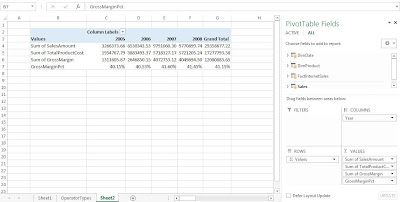Fig. 5: PivotTable with GrossMarginPct

#### When to Use Calculated Columns and Measures (Fields)

So when do we use a calculated column and in other cases, a calculated field (or measure)? (When do we get to eat Jell-O?) In most situations, it would depend on the computation of choice. For example, there are several situations to use calculated columns:

• When we want to evaluate an expression which is confined to the current row,
• The results will be displayed in the columns or rows of the PivotTable, and not the values,
• Or when we categorize text or numbers (categorical variables)

Situations where we would use calculated fields occur when we would like to display the results in the PivotTable Values section. Each cell in the Values section will change based on the context of the rows and columns in the PivotTable selections. If we changed the rows in Figure 5 to include the ModelName, then the calculationed field would reflect the change, and show the GrossMarginPct stratified by ModelName (Figure 6).Fig. 6: PivotTable with ModelName Stratifying the Calculated Field

#### Cross-Referencing

We can use calculated columns in our DAX expressions when creating calculated fields. Also, the reverse can be done as well. However counter-intuitive, a calculated column can reference calculated fields, and this confines the calculated field to the context of the current row. Therefore, the calculated field transforms into a calculated column, and will not be influenced by user changes in context on the PivotTable.

Here we have explored the similarities and (for the most part) differences between calculated columns and fields through DAX. Understanding these concepts are important in becoming proficient at DAX, as well being able to manipulate data efficiently.

Wayne

## Sunday, August 18, 2013

### P3.1: Beginning DAX 1

Hi Readers, this post will introduce the DAX language. As usual, we will use Chapter 3 Sales Example of the companion material of the Ferrari PowerPivot book. Let us get started.

### Fundamentals of DAX

First, a little background. DAX stands for Data Analysis eXpressions language. This PowerPivot syntax is similar to Excel expressions, but also allows users to handle data stored in different tables in more advanced calculations. Therefore, while Excel uses table coordinates such as A1, or C3, DAX uses tables and columns as coordinates. Post 1.6 touched upon the notion of row and column oriented databases. Clearly, DAX is column oriented, and can pull a value for a column in a single row, the entire table, or some subset in between.

We will cover DAX syntax, data types handled by DAX, basic operators, and how to refer to tables and columns in PowerPivot in this post. Here we go!

### DAX Syntax

Let us start by an example. Opening the PowerPivot window, we observe the Sales table with columns SalesAmount and TotalProductCost towards the end.Fig. 1: Sales Table in PowerPivot
Subtracting TotalProductCost from the SalesAmount gives us the margin. Type the following formula for the GrossMargin:
=Sales[SalesAmount]-Sales[TotalProductCost]Fig. 2: GrossMargin Column Added
We can see from the formula (a calculated column) that we refer to the table name and the column name for this DAX expression, which handles numeric values and returns numerical values. This is basic DAX syntax.

### DAX Data Types

DAX can handle numerical values, such as:

• Integer
• Real
• Currency
• Date (or datetime)
• True/False (Boolean)
• String
• BLOB (Binary Large OBject)

PowerPivot has a great type-handling system; we can use DAX expressions and the resulting type is based on the type of terms used in the expressions. So if a term in the expression is a date, then the result would be a date, if an integer, then the result would be an integer. This is called operator overloading. For example, we can add a week to the Date column by adding seven to create a DatePlusOneWeek column.
=Sales[Date] + 7Fig. 3: DatePlusOneWeek Column Calculation
The DAX expression adds 7 days (one week), to the Date column and returns the Date a week later, in DatePlusOneWeek. June 1, 2008 was transformed into June 8, 2008, as a date numeric type.

PowerPivot will automatically convert numbers into strings and in reverse when the operator requires it. So when we have the expression

= 5 + 4
and replace the operator with & to concatenate strings, the 5 and 4 will be converted into strings. The result of
= 5 & 4
is "54" (the " " indicates a string.) In reverse, when we have strings  "5" and "4" being added together:
= "5" + "4"
we get 9. Using the operator to determine the type of the resulting value is convenient, but we will encounter handling errors in later posts.

Considerations of Specific Data types:

Date
PowerPivot stores datetime as a floating point number. In fact, the floating point number corresponds to the number of days starting from December 30, 1899, and the decimal represents the fraction of the day. The seconds, minutes, and hours in a day are converted into decimal fractions. If we evaluate this idea by:
= NOW() + 1
we would have an expression which adds one to the floating point. Thus it adds one day to today and we get essentially the date for tomorrow (with the same hours, minutes, and seconds.)

True and False
True and False represent logical conditions, and is usually called a Boolean data type. For example, the expression
=Sales[TotalProductCost] >= Sales[SalesAmount]
would return True if the TotalProductCost of the row was greater or equal to the SalesAmount, and False if the it was less.

### Dax Operators

Figure 4 below shows the different DAX operators and examples. We have seen many before.Fig. 4: DAX Operator Types

### DAX Values

The values used in Figure 4, such as "USA" or 0, are called literals and is straightforward. However, when referencing column names in the format:
'Table'[Column]
we usually can omit the quotation marks if the table name has no special characters or spaces to:
Table[Column]
We also can use the column name by itself, which is always enclosed in square brackets:
[Column]
and the expression will find the referenced column name in the current table.
For the sake of readability, and to prevent mistakes, use the table name in conjunction with the column name.

### Note on IntelliSense:

When we type into the formula box in PowerPivot, a helpful window appears, listing the names of all the function names and references. For example, if we type in
=[
to begin a column name, a listing of all the column names will appear.Fig. 5: IntelliSense Displaying the Possible Column Names
We will continue to explore DAX in the next post, P3.2.

Wayne

## Saturday, August 17, 2013

### P2.3: Computing Complex Aggregations in PowerPivot: Distinct Count & Refreshing the Data Model

This post will describe how to calculate a complex aggregation such as a distinct count of products in PowerPivot. We will be using the data model created in the previous post, from the companion material of the Ferrari PowerPivot book.

### Computing Distinct Count

Calculated fields have an useful feature that enables us to compute complex aggregations. In the last post we calculated the gross margin and also the gross percentage of sales using calculated column and field. Today we would like to determine the number of products in each Color.

Let us begin by creating a simple PivotTable showing the SalesAmount by Color of products.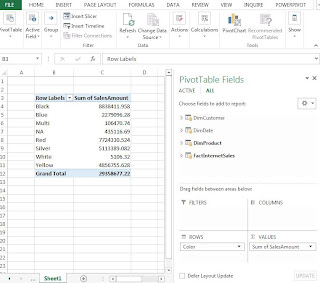Fig. 1: Sum of SalesAmount by Color of Product
We want to count the number of products, not the number sold under each Color; whether a particular product sold 8,000 units or only 1 unit, the count would only be 1 for that same product (hence distinct count.)

If we add the ProductKey under the DimProduct table to the PivotTable, we can see that the result is not what we were looking for.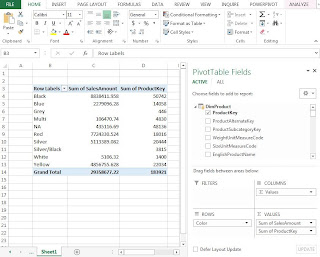Fig. 2: PivotTable with Sum of ProductKey
From the numbers and the column header, the column is displaying the sum of the product keys. It is logical that Excel took the sum because the values are integers. However we want the sum of the number of unique product keys. We can change the method Excel aggregates the data, by changing the settings of ProductKey in the Values area. Click the drop-down arrow and select the Value Field Settings button.Fig. 3: Value Field Settings Button
In the Value Field Settings window, we can select an attractive option, which also happens to be called Distinct Count. Note that the Custom Name of the column changes to "Distinct Count of ProductKey".Fig. 4: Value Field Settings- Distinct Count Option
Now let us observe the new PivotTable showing the proper distinct count. The two red arrows point to rows with counted products but no sales. If we want to count products responsible for sales, we should only count those products that were actually sold.Fig. 5: PivotTable with Corrected Distinct Count
The correct field to display in the PivotTable is the ProductKey under the FactInternetSales table, because the table has only product keys of products that were sold. This highlights how easily we could choose the wrong field to aggregate, especially for the computation of distinct count. The PivotTable with correct fields is shown below.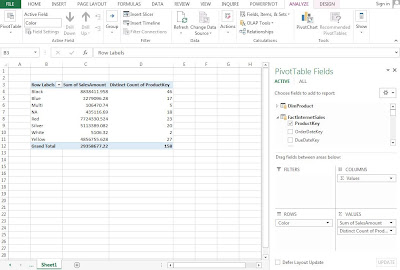Fig. 6: PivotTable with Proper Distinct Count

### Note on Refreshing the Data Model:

We have seen in P2.1 that we can add data to the data model in PowerPivot without going through Excel. Data models are dynamic, and thus, they change constantly as columns or fields are calculated. Refresh the data model by clicking the Refresh button in the Home tab of PowerPivot. It will give the option of refreshing the current table or all of the tables in PowerPivot, as highlighted in Figure 7.Fig. 7: Refresh Button
When PowerPivot refreshes tables, it will gather the data again from the databases and update the tables and the calculated columns accordingly. Calculated fields are not stored in the data model so they are recalculated as required. Because we work with data sets not numbering in the millions of rows, the refresh process is quick. However, it can take some amount of time when many millions of rows are read again from the database. This is code we will explore later on, which will update the tables upon opening, or clicking a certain button.

Wayne

### P2.2: Creating Calculated Columns and Calculated Fields in PowerPivot

This post will explore calculated columns and fields in PowerPivot, using the sample database from the Ferrari PowerPivot book. We will continue to use the PivotTable and data model from the previous post.

### Creating a Calculated Column

From the previous post, we created a PivotTable depicting the SalesAmount by Color, and CalendarYear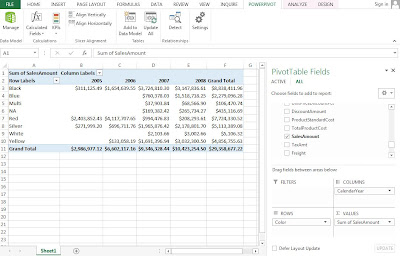Fig. 1: SalesAmount by Color by CalendarYear PivotTable
To understand the profitability from the sales, we account at least for the product cost as well. So the TotalProductCost column needs to be added from the FactInternetSales table.Fig. 2: SalesAmount with TotalProductCost PivotTable

The TotalProductCost column was added beside each SalesAmount column in the PivotTable shown in Figure 2. For certain reports arranging columns side by side is better, but for readability for this report, we could like to position the fields in rows resembling the subtraction to obtain the profit data. So drag the Σ Values to the Rows area, as in Figure 3.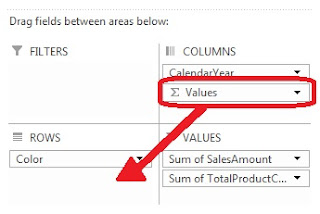Fig. 3: Dragging the Σ Values to Rows Area
The PivotTable is now easier to read and set up for the calculated column.Fig. 4: Revised SalesAmount and TotalProductCost PivotTable
The FactInternetSales table has no gross margin column, which is the difference between SalesAmount and TotalProductCost. So we will add a column called GrossMargin in PowerPivot as a calculated column, which extends the content of a table and is stored in the data model. First, navigate to the FactInternetSales table in the PowerPivot window. In the Design tab, click the Add button which will add a new column to the end of the table.Fig. 5: Add Column Button
Now we use simple DAX language to calculate the GrossMargin column. Though DAX syntax can be more intensive, the formula is very straight forward. Type in:
= FactInternetSales[SalesAmount] - FactInternetSales[TotalProductCost]
as shown in the Figure 6 below. It subtracts the TotalProductCost from the SalesAmount for the GrossMargin column, and it is now in the data model. The column can be renamed by right-clicking the column header and selecting Rename Column.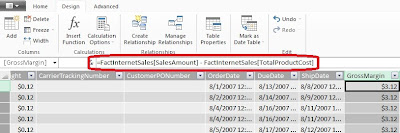Fig. 6: GrossMargin Formula
Let us add the GrossMargin column to the Values area in the PivotTable. We can find it in the FactInternetSales table where we created it in PowerPivot. It would go in the Values area.Fig. 7: PivotTable with GrossMargin
We can use DAX to solve more complex calculations, which we will explore in future posts. However with this simple DAX formula we have created a calculated column and displayed it on the PivotTable.

### Creating a Calculated Field

A calculated field, also known as measures, are computed at an aggregate level, compared to calculated columns, which are computed for every row. For example, let us compute the gross margin as a percentage of the total sales amount.

Add a new column in PowerPivot to the FactInternetSales table which calculates the gross margin percentage. We might enter this as the formula for GrossMarginPct:
=FactInternetSales[GrossMargin] / FactInternetSales[SalesAmount]Fig. 8: Formula for GrossMarginPct
Then display the resulting GrossMarginPct in the PivotTable under the Values area.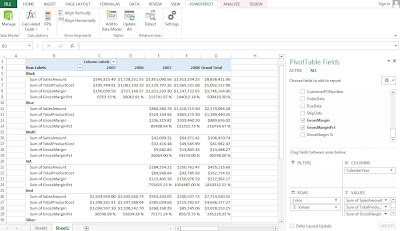Fig. 9: PivotTable Including GrossMarginPct
Wait! The GrossMarginPct numbers look quite large and not right at all. In fact, the formula took the summation of all the percentages and displayed the sum, which is not what we wanted (this is the incorrect way to formulate this GrossMarginPct calculated field.) We can remove the GrossMarginPct from the PivotTable.

The correct way to obtain the GrossMargin percentage as a calculated field is to click on the Calculated Fields button in the PowerPivot tab.Fig. 10: Calculated Fields Button
Name the new field GrossMargin%, and for the formula, type in:
=SUM(FactInternetSales[GrossMargin]) / SUM(FactInternetSales[SalesAmount])
as shown down below.Fig. 11: Creating the GrossMargin% Field
The syntax for the calculated field is similar to the calculated column formula; it just has an additional SUM function. This will be explained in later posts describing the DAX language. In the window, we are given options at the bottom to format the output of the calculation, and since it is a percentage, select the Percentage format. After we click OK, the calculated field, GrossMargin%, is added to the data model and is available to complete the PivotTable. Select it and confirm that it is in the Values area.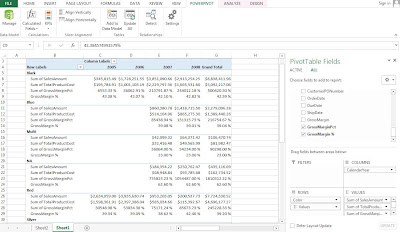Fig. 12: Revised PivotTable with GrossMargin%
Now these percentages look much more reasonable than the previous gross margin calculation, because it took the aggregate of the SalesAmount and the TotalProductionCost. We take a deeper look at calculated columns and fields later in P3.2.

#### Note:

The calculated field will be available to the PivotTable or report that we create using the data model. Think of it like this: we are building a data model, not just a report. The data model can be used for many other different reports or PivotTables, so as we transition into a good data modeler, we become better report creators.